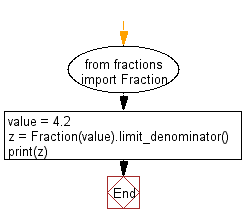﻿ Python Math: Convert a float to ratio - w3resource# Python Math: Convert a float to ratio

## Python Math: Exercise-82 with Solution

Write a Python program to convert a float to ratio.

Sample Solution:-

Python Code:

``````from fractions import Fraction
value = 4.2
print(Fraction(value).limit_denominator())
```
```

Sample Output:

```21/5
```

Flowchart:Python Code Editor:

Have another way to solve this solution? Contribute your code (and comments) through Disqus.

What is the difficulty level of this exercise?

Test your Python skills with w3resource's quiz

﻿

## Python: Tips of the Day

Python: Unpack a Tuple

```>>> items = (0, 'b', 'one', 10,  11, 'zero')
>>> a, b, c, d, e, f = items
>>> print(f)
zero
>>> a, *b, c = items
>>> print(b)
['b', 'one', 10, 11]
>>> *_, a, b = items
>>> print(a)
11
```